# Important Questions on Kinematics for JEE Advanced , Jee Main and NEET

Question 1
A particle moves in a straight line according to the relation
$x=t^3-4t^2+3t$
Find the acceleration of the particle at displacement equal to zero.
a. (-8,-2,10)
b. (-1,-2,10)
c. (8,2,10)
d. (1,2,10)

Question 2
A body starts at initial velocity $v_0$ in a straight line with acceleration as shown below in the graph.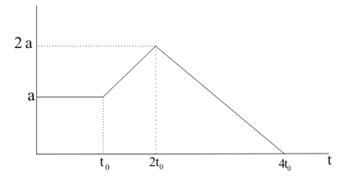Find the maximum velocity reached.
a. $v_0+4at_0$
b. $v_0+ \frac {9}{2}(at_0)$
c. $v_0 - \frac {9}{2}(at_0)$
d. $v_0 - 4at_0$

Question 3
A particle moves in a straight line with acceleration described by equation given below
$a=mx- \frac {v_{0}^{2}}{x_0}$
If the initial velocity and displacement are $(v_0, 0)$ and at any time $t_0$ velocity and displacement are $(0, x_0)$ the value of constant m is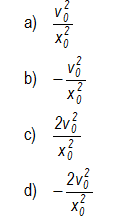Question 4
A boat moves with the stream of water from point A and B and it return back with the same speed. Velocity of boat relative to water is $\eta$ times the velocity of the water. Velocity of the water is 1m/sec. Find out the average speed in the whole iternary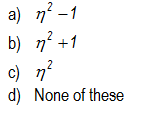Question 5
A body is freely falling under the action of gravity. It covers half the total distance in the last second of its fall. If it falls for n second, then value of n is
a. $2$
b. $2 + \sqrt {2}$
c. $3$
d. $2 - \sqrt {2}$

Question 7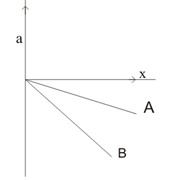Two particle A and B start with the same velocity $v=v_0$ at x=0.They are accelerated per the graph shown above. Which particle has the maximum magnitude of the velocity at $x=x_0$
a. A
b. B
c. A & B will have same velocity
d. None of the above

Question 8
Distance and displacement of a moving object have same magnitude when
a. When object moves in circular motion
b. When object moves along a zig-zag path
c. When object moves along straight line and always moves along the same direction
d. When it moves along straight line but the distance is not always same

Question 9
The greatest possible acceleration or deceleration a train may have is a and its maximum speed is v. Find the maximum time in which the train can get from one station to the next if the total distance is s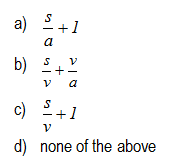Question 10
Displacement(y) of the particle is given by
$y=2t+t^2-2t^3$
the velocity of the particle when acceleration is zero is given by
a. $\frac {5}{2}$
b. $\frac {9}{4}$
c. $\frac {13}{6}$
d. $\frac {17}{8}$

Question 11
A particle is set in motion at t=0 such that velocity varies as $v= v_0(1 - \frac {t}{2})$ where $v_0$ is a positive constant. Find the distance, displacement covered by the particle in first 3 sec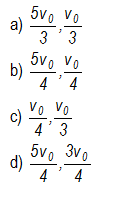Question 12
The velocity, displacement, acceleration of a particle in one dimensional motion is given as
$v_1,x_1,a_1$ at $t=t_0$
$v_2,x_2,a_2$ at $t=t_0 + \Delta t$
which of the following is correct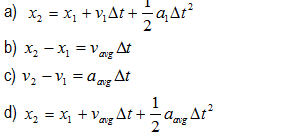Question 13
The displacement time equation for a particle in linear motion is given as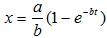which of the following option is correct
a. The velocity and acceleration of the particle at t=0 is $a$ and $-ab$ respectively
b. The velocity will be decreasing as the time increases
c. The displacement of the particle will fall between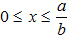d. The maximum acceleration in the motion is $-ab$

Question 14.
Consider the figure given below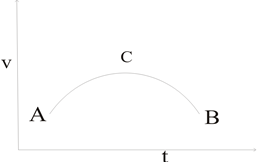a.Acceleration is positive during A to C and negative during C to B
b.Acceleration is maximum at C
c.Velocity is maximum at C
d. Average acceleration is zero during the journey

Question 15.
Which one of the following statement(s) is true?
a.A body moving with uniform speed can have variable velocity
b.A body moving with uniform velocity can have variable speed
c.Average velocity is always equal to instantaneous velocity
d.x-t graph can be a straight line parallel to position axis

Question 16.
Which one of the following statement is correct?
a. A body has constant speed but varying velocity
b. A body has constant speed but varying acceleration
c. A body having constant speed cannot have acceleration
d. None of the above

Question 17.
A body moves along a semicircular track of Radius R. Which of the following statement is true
a. Displacement of the body is $2R$
b. Distance travelled by the body is $\pi R$
c. Displacement of the body is $\pi R$
d. none of the above

Question 18.
Which of the following is false
a. The speed of the particle at any instant is given by the slope of the displacement-time graph
b. The distance moved by the particle in a time interval from t1 to t2 is given by the area under the velocity -time graph during that time interval
c. Magnitude of the acceleration of the particle at any instant is given by the slope of the velocity time graph
d. none of the above are true

Question 19.
A particle is going moving along x-axis. Which of the following statement is false
a. At time t1 (dx/dt)t=t1=0,then (d2x/dt2)t=t1=0
b. At time t1 (dx/dt)t=t1 < 0 then the particle is directed towards origin
c. If the velocity is zero for a time interval, the acceleration is zero at any instant within the time interval.
d. At time t1 (d2x/dt2)t=t1 < 0 then the particle is directed towards origin

Question 20.
A particle starts at time t=0 from x=0 along the positive x-axis with constant speed v .After time t,it return back towards the origin with the speed 2v and reaches the origin in t/2 sec .Which of the following is true for the whole process
a. Average velocity is zero for the whole process
b. Average speed is $\frac {4v}{3}$ for the whole process
c. Displacement at time t is equal to vt d. Displacement at time $\frac {3t}{2}$ is $2vt$

Question 21.
A car, starting from rest is accelerated at constant rate a until it attains speed v. It is then retarded at a constant rate b until it comes to rest. which of the following is true
a. the average speed for the whole motion is $\frac {av}{2b}$
b. the average speed for the whole motion is $\frac {v}{2}$
c. Total time taken for the journey is $v(\frac {1}{a}+\frac {1}{b})$
d. none of the above

Paragraph type question
(A) A Man X drops a stone from the fifteen floor of the building .A Man Y ascending in an elevator at a constant speed v=10 m/s passed the Fifteen floor just as the stone is released
Question 22.
Find the position, velocity of the stone as seen by the Man X at time t=2 sec
a. (19m ,19m/s)
b. (19.6m,19.6m/s)
c. (10m,10m/s)
d (11m,12m/s)

Question 23
Find the position, velocity of the stone relative to Man Y at 3 sec
a. (39m, 70m/s)
b. (19m, 70m/s)
c. (70m, 39 m/s)
d. (14 m, 29m/s)

Question 24
Find the acceleration of the stone with respect to Man X and Y.
a. (9.8 m/s2, 9.8m/s2)
b. (9 m/s2, 10 m/s2)
c. (10 m/s2, 10m/s2)
d. None of these

(C) A gun is mounted on a train roof .Train is travelling with the velocity $v$ in north direction. A car is also moving on a parallel track with train with velocity $w$ in north direction. Two bullet are fired from the muzzle of the gun .Take north as positive and south as negative
Question 25
Bullet one is fired in the north direction with the muzzle velocity $u$. Find the velocity of the bullet as seen from the observer on the earth
a. $u+v$
b. $u-v$
c. $u$
d. $v$

Question 26
Find the velocity of the bullet as seen from the observer on the moving car
a. $u+v-w$
b. $u-v-w$
c. $u$
d. $v$

Question 27
Bullet one is fired in the south direction with the muzzle velocity u. Find the velocity of the bullet as seen from the observer on the earth
a. $u+v$
b. $v-u$
c. $u$
d. $v$

Question 28
Find the velocity of the second bullet as seen from the observer on the moving car
a. u+v-w
b. v-u-w
c. u
d. v

(D) A train is moving in the west direction with a velocity 15m/s.A monkey runs on the roof of the train against its motion with a velocity  5m/s with respect to train .Take the motion along west as positive
Question 29
Velocity of train relative to its driver
a. 0
b. 15 m/s
c. -15 m/s
d. 20 m/s

Question 30
What is the velocity of train with respect to monkey
a. 5m/s
b -5 m/s
c. 15 m/s
d -15 m/s

Question 31
Find the velocty of ground with respect to monkey
a. 5 m/s
b. -5 m/s
c. 10 m/s
d. -10 m/s

Question 32
Water drips from a faucet at a uniform rate of m drops per second. Find the distance x between the two adjacent drops as a function of time t that the trailing drop has been in motion.
a. $x = g\frac {t}{m} + g \frac {1}{2m^2}$
b. $x = g \frac {1}{2m^2}$
c. $x = g \frac {1}{4m^2}$
d. $x=g\frac {t}{2m} + g \frac {1}{2m^2}$

Tricky Kinematics Questions
Question 33
A lift is coming from 8th floor and is just about to reach 4th floor. Taking ground floor as origin and positive direction upwards for all quantities, which one of the following is correct?
(a) x < 0, v < 0, a > 0
(b) x > 0, v < 0, a < 0
(c) x > 0, v < 0, a > 0
(d) x > 0, v > 0, a < 0

Question 34
In one dimensional motion, instantaneous speed v satisfies $0 \leq v < v_0$.
(a) The displacement in time T must always take non-negative values.
(b) The displacement x in time T satisfies $�v_0 T < x < v_0 T$.
(c) The acceleration is always a non-negative number.
(d) The motion has no turning points

Question 35
For the one-dimensional motion, described by $x = t�sint$
(a) x (t) > 0 for all t > 0.
(b) v (t) > 0 for all t > 0.
(c) a (t) > 0 for all t > 0.
(d) v (t) lies between 0 and 2